Next: Spin-1/2 Paramagnetism Up: Applications of Statistical Thermodynamics Previous: Introduction

# Canonical Probability Distribution

We have gained some understanding of the macroscopic properties of the air in a classroom (say). For instance, we know something about its internal energy and specific heat capacity. How can we obtain information about the statistical properties of the molecules that make up this air? Consider a specific molecule. It constantly collides with its immediate neighbor molecules, and occasionally bounces off the walls of the room. These interactions inform'' it about the macroscopic state of the air, such as its temperature, pressure, and volume. The statistical distribution of the molecule over its own particular microstates must be consistent with this macrostate. In other words, if we have a large group of such molecules with similar statistical distributions then they must be equivalent to air with the appropriate macroscopic properties. So, it ought to be possible to calculate the probability distribution of the molecule over its microstates from a knowledge of these macroscopic properties.

We can think of the interaction of a molecule with the air in a classroom as analogous to the interaction of a small system,, in thermal contact with a heat reservoir,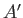. The air acts like a heat reservoir because its energy fluctuations due to interactions with the molecule are far too small to affect any of its macroscopic parameters. Let us determine the probability,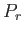, of finding systemin one particular microstate,, of energy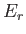, when it is thermal equilibrium with the heat reservoir,.

As usual, we assume fairly weak interaction betweenand, so that the energies of these two systems are additive. The energy ofis not known at this stage. In fact, only the total energy of the combined system,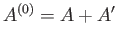, is known. Suppose that the total energy lies in the range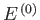to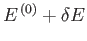. The overall energy is constant in time, because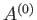is assumed to be an isolated system, so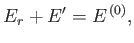(7.1)

where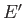denotes the energy of the reservoir. Let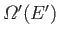be the number of microstates accessible to the reservoir when its energy lies in the rangeto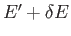. Clearly, if systemhas an energythen the reservoirmust have an energy close to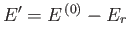. Hence, becauseis in one definite state (i.e., state), and the total number of states accessible tois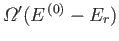, it follows that the total number of states accessible to the combined system is simply. The principle of equal a priori probabilities tells us the probability of occurrence of a particular situation is proportional to the number of accessible microstates. Thus,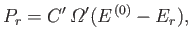(7.2)

where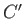is a constant of proportionality that is independent of. This constant can be determined by the normalization condition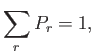(7.3)

where the sum is over all possible states of system, irrespective of their energy.

Let us now make use of the fact that systemis far smaller than system. It follows that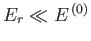, so the slowly-varying logarithm ofcan be Taylor expanded about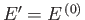. Thus,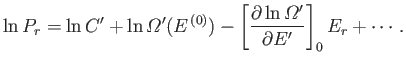(7.4)

Note that we must expand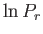, rather thanitself, because the latter function varies so rapidly with energy that the radius of convergence of its Taylor series is too small for the series to be of any practical use. The higher-order terms in Equation (7.4) can be safely neglected, because. Now, the derivative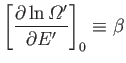(7.5)

is evaluated at the fixed energy, and is, thus, a constant, independent of the energy,, of. In fact, we know, from Chapter 5, that this derivative is just the temperature parameter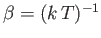characterizing the heat reservoir. Here,is the absolute temperature of the reservoir. Hence, Equation (7.4) becomes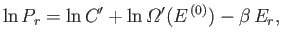(7.6)

giving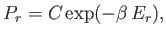(7.7)

where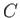is a constant independent of. The parameteris determined by the normalization condition, which gives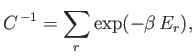(7.8)

so that the distribution becomes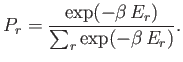(7.9)

This distribution is known as the canonical probability distribution (it is also sometimes called the Boltzmann probability distribution), and is an extremely important result in statistical physics.

The canonical distribution often causes confusion. People who are familiar with the principle of equal a priori probabilities, which says that all microstates are equally probable, are understandably surprised when they come across the canonical distribution, which says that high energy microstates are markedly less probable then low energy states. However, there is no need for any confusion. The principle of equal a priori probabilities applies to the whole system, whereas the canonical distribution only applies to a small part of the system. The two results are perfectly consistent. If the small system is in a microstate with a comparatively high energy,, then the remainder of the system (i.e., the reservoir) has a slightly lower energy,, than usual (because the overall energy is fixed). The number of accessible microstates of the reservoir is a very strongly increasing function of its energy. It follows that if the small system has a high energy then significantly less states than usual are accessible to the reservoir, so the number of microstates accessible to the overall system is reduced, and, hence, the configuration is comparatively unlikely. The strong increase in the number of accessible microstates of the reservoir with increasinggives rise to the strong (i.e., exponential) decrease in the likelihood of a stateof the small system with increasing. The exponential factor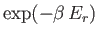is called the Boltzmann factor.

The canonical distribution gives the probability of finding the small systemin one particular stateof energy. The probability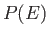thathas an energy in the small range between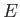and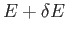is just the sum of all the probabilities of the states that lie in this range. However, because each of these states has approximately the same Boltzmann factor, this sum can be written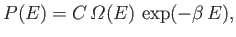(7.10)

where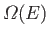is the number of microstates ofwhose energies lie in the appropriate range. Suppose that systemis itself a large system, but still very much smaller than system. For a large system, we expectto be a very rapidly increasing function of energy, so the probabilityis the product of a rapidly increasing function of, and another rapidly decreasing function (i.e., the Boltzmann factor). This gives a sharp maximum ofat some particular value of the energy. As systembecomes larger, this maximum becomes sharper. Eventually, the maximum becomes so sharp that the energy of systemis almost bound to lie at the most probable energy. As usual, the most probable energy is evaluated by looking for the maximum of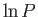, so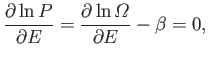(7.11)

giving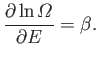(7.12)

Of course, this corresponds to the situation in which the temperature ofis the same as that of the reservoir. This is a result that we have seen before. (See Chapter 5.) Note, however, that the canonical distribution is applicable no matter how small systemis, so it is a far more general result than any that we have previously obtained.Next: Spin-1/2 Paramagnetism Up: Applications of Statistical Thermodynamics Previous: Introduction
Richard Fitzpatrick 2016-01-25